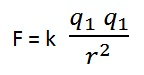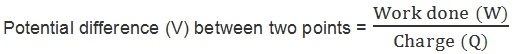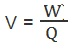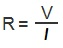Study Materials:
CBSE NOTES ⇒ Class 10th ⇒ Science ⇒ 12. Electricity

## 12. Electricity

### Introduction

12. Electricity

Frictional electricity: The electricity developed by rubbing or friction is called frictional electricity.

Electric charge: There are two types of electric charge,

1. Positive charge: Charge acquired by a glass rod when rubbed with silk is called positive charge.

2. Negative charge: Charge acquired by a ebonite rod when rubbed with wool is called negative charge.

• Positive charge is produced due to less electrons.
• Negative charge produced due to more electrons.

Fundamental law of electrostatics:

• Like charges repel each other.
• Unlike charge attract each other.

Statics electricity: Statics electricity deals with the electric charges at rest.

Current electricity: current electricity deals with the electric charges in motion.

Electric Current And Charge:

When the electric charge flows through a conductor, we say that there is an electric current in the conductor.

In other words: An electric current is a flow of a electric charge.

Electric current is expressed by the amount of charge flowing through a particular area in unit time.

• Electric current flows throught a wire/conductor.
• Electric current is a scalar quantity.

Flowing/moving of electrons:

The electrons are repelled by the negative charge at the negative terminal of the battery and attracted by the positive charge at the positive terminal. Therefore the electrons drift away from the negative terminal and toward the positive terminal. When electrons reach the positive terminal a chemical reaction transfers them across the battery and back to the negative terminal.

Conductor:

A substances which allows the passage of electric charge throught it easily, is called conductor. Example: copper, silver, aluminium etc.

• Good conductors have low resistance to the flow of current.
• Bad conductors have high resistance to the flow of current.

Insulator: A substance which does not allow electric current to flow through it is called insulator. Example- rubber, plastic ebonite, glass etc.

Cunductivity: Conductivity is a property of conductor by which it allow the passage electric charge throught it.

Supercunductivity: Superconductivity is a phenomenon of exactly zero electrical resistance at very low temperature.

Coulomb's law: The force of attraction or repulsion between two point charges are directly proportional to the product (q1q2) of the two charges and inversely proportional to the square of the the distance(r) between them.

Mathematically,

F ∝ q1q2          ......................... (i)

F ∝ 1/ r2          ..........................(ii)k is constant value, but the value of K depends on the nature of the medium between the two charges.

Charges in vacuum of K = 9 × 109 Nm2/C2

Electric Circuit:

A continuous and closed path of an electric current is called an electric circuit.

The flow of the electricity:

Electrons constitute the flow of charge. electric current was considered to be the flow of positive charges and the direction of flow of positive charges was taken to be the direction of electric current.

If a net charge Q, flows across any cross-section of a conductor in
time t, then the current I, through the cross-section is;

I = Q/t

The SI unit of electric charge is coulomb (C), which is equivalent to the charge contained in nearly 6 × 1018 electrons.

Coulomb: It is the SI unit of charge, which is equivalent to the charge contained in nearly 6 × 1018 electrons.

Charge on an electron = -1.6 × 10-19 coulomb (C).

Charge on a proton = 1.6 × 10-19 coulomb (C).

Law of conservation of charge: Electric charges can neither be created nor destroyed, they can only be transferred from one body to another body.

Ampere: It is the SI unit of current. When one coulomb of charge flows in one second it is called one Ampere of current.

1A = 1C/1s;

• Small quantities of current are expressed in milliampere (1 mA = 10-3 A) or microampere (1 μA = 10-6 A).

The electric current flows in the circuit from the positive terminal of the cell to the negative terminal of the cell through the bulb and ammeter.

Ammeter: It is a device to measure electric current in a circuit. It is always connected in series in a circuit.

Galvanometer: It is a device to detect current in an electric circuit.

Conventional current: Conventionally, the direction of motion of positive charges is taken as the direction of current. The direction of conventional current is opposite to the flow of electron.

Direction in which an electric current is considered to flow in a circuit. By convention, the direction is that in which positive-charge carriers would flow - from the positive terminal of a cell to its negative terminal. It is opposite in direction to the flow of electrons.

Electrostatic potential:

Electrostatic potential at any point in an electric field is defined as the amount of work done in bringing a unit positive charge of one coulomb from infinity to that point. Its unit is volt.

Potential Difference:

The electrons move only if there is a difference of electric pressure, this difference in electric pressure is called the potential difference – along the conductor.

• This difference of potential may be produced by a battery, consisting of one or more electric cells.
• The chemical action within a cell generates the potential difference across the terminals of the cell, even when no current is drawn from it.
• When the cell is connected to a conducting circuit element, the potential difference sets the charges in motion in the conductor and produces an electric current. In order to maintain the current in a given electric circuit, the cell has to expend its chemical energy stored in it.

Potential difference between two points:

the electric potential difference between two points in an electric circuit carrying some current as the work done to move a unit charge from one point to the other.Or• The SI unit of electric potential difference is volt (V), named after Alessandro Volta (1745–1827), an Italian physicist.

Voltmeter:

The potential difference is measured by means of an instrument called
the voltmeter.

One volt potential difference:

One volt is the potential difference between two points in a current carrying conductor when 1 joule of work is done to move a charge of 1 coulomb from one point to the other.Connection of voltmeter:

The voltmeter is always connected in parallel across the points between which the potential difference is to be measured.

Cells Or Battery: It is a device which helps to maintain the potential difference between two points of conductor.

Electromotive force (EMF): It is a force applied by cell or battery to produce electric current through a wire.

##### Ohm's Law:

The electric current flowing through a metallic wire is directly proportional to the potential difference V, across its ends provided its temperature remains the same. This is called Ohm’s law.

According to this law.

V ∝ I            or  V = RI

see this volt-current graphif potential difference is increased then electric current also increases.

And if potential difference is decreases then electric current also decreases.

Resistance:

It is the property of a conductor to resist the flow of charges through it.

Using ohm law:

Resistance = Potential difference/Current• SI units of resistance is Ohm(Ω).
• V/I = R, which is a constant.

1 Ohm of resistance: if the potential difference across the two ends of a conductor is 1 V and the current through it is 1 A, then the resistance R, of the conductor is 1 Ω.• The current through a resistor is inversely proportional to its resistance.
• If the resistance is doubled the current gets halved.
• In many practical cases it is necessary to increase or decrease the current in an electric circuit.

Variable resistance: A component used to regulate current without changing the voltage source is called variable resistance.

Rheostat: It is a device which is often used to change the resistance in the electric circuit.

Resistor: A conductor having some appreciable resistance is called a resistor.

Some points:

• Certain components offer an easy path for the flow of electric current while the others resist the flow.
• motion of electrons in an electric circuit constitutes an electric current.
• The electrons, however, are not completely free to move within a
conductor.
• They are restrained by the attraction of the atoms among which they move.
• motion of electrons through a conductor is retarded by its resistance.

Good conductor: A component of a given size that offers a low
resistance is a good conductor.

Poor conductor: A component of identical size that offers a higher resistance is a poor conductor.

Resistance of a conductor depends on followings factors:

(i) On Length of conductor.

(ii) On Area of cross-section of conductor.

(iii) On the nature of conductor's materail.

Relation among Length, Area and Resistance:

Resistance of a uniform metallic conductor is directly proportional to its length (l) and inversely proportional to the area of cross-section (A).

This gives:

R ∝ l                              ..................  (i)

and    R ∝ l/A                           ................... (ii)

R ∝ l/A                          using (i) and (ii)

or,where ρ (rho) is a constant of proportionality and is called the electrical
resistivity of the material of the conductor.

The SI unit of resistivity is Ω m.

Resistivity: Resistivity is a characteristic property of the material. S. I unit of resistivity is ohm (Ω). It is denoted by p.

• The metals and alloys have very low resistivity in the range of 10-8 Ω m to 10-6 Ω m.They are good conductors of electricity.
• Insulators like rubber and glass have resistivity of the order of 1012 to 1017 Ω m.
• Both the resistance and resistivity of a material vary (परिवर्तित) with temperature.

Charactristics of alloy:

(i) The resistivity of an alloy is generally higher than that of its constituent metals.

(ii) Alloys do not oxidise (burn) readily at high temperatures.

(iii) They have high melting point.

Therefore, alloys are commonly used in electrical heating devices, like electric iron, toasters etc. Tungsten is used almost exclusively for filaments of electric bulbs, whereas copper and aluminium are generally used for electrical transmission lines.

Other Pages of this Chapter:

Important Study materials for classes 06, 07, 08,09,10, 11 and 12. Like CBSE Notes, Notes for Science, Notes for maths, Notes for Social Science, Notes for Accountancy, Notes for Economics, Notes for political Science, Noes for History, Notes For Bussiness Study, Physical Educations, Sample Papers, Test Papers, Mock Test Papers, Support Materials and Books.Mathematics Class - 11th

NCERT Maths book for CBSE Students.

books

## Study Materials List:

##### CBSE NOTES ⇒ Class 10th ⇒ Science
1. Chemical Reactions and Equations
2. Acids, Bases and Salts
3. Metals and Non-metals
4. Carbon and its Compounds
5. Periodic Classification of Elements
6. Life Processes
7. Control and Coordination
8. How do Organisms Reproduce
9. Heredity and Evolution
10. Light-Reflection and Refraction
11. Human Eye and Colourful World
12. Electricity
13. Magnetic Effects of Electric Current
14. Sources of Energy
15. Our Environment
16. Management of Natural Resources

New Books

See More ...